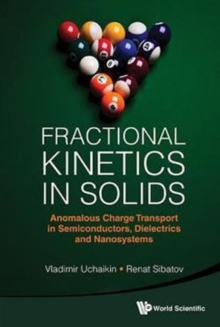Supporting your high street Find out how »
• My Account# Fractional Kinetics In Solids: Anomalous Charge Transport In Semiconductors, Dielectrics And Nanosystems Hardback

#### Description

The standard (Markovian) transport model based on the Boltzmann equation cannot describe some non-equilibrium processes called anomalous that take place in many disordered solids.

Causes of anomality lie in non-uniformly scaled (fractal) spatial heterogeneities, in which particle trajectories take cluster form.

Furthermore, particles can be located in some domains of small sizes (traps) for a long time.

Estimations show that path length and waiting time distributions are often characterized by heavy tails of the power law type.

This behavior allows the introduction of time and space derivatives of fractional orders.

Distinction of path length distribution from exponential is interpreted as a consequence of media fractality, and analogous property of waiting time distribution as a presence of memory.In this book, a novel approach using equations with derivatives of fractional orders is applied to describe anomalous transport and relaxation in disordered semiconductors, dielectrics and quantum dot systems.

A relationship between the self-similarity of transport, the Levy stable limiting distributions and the kinetic equations with fractional derivatives is established.

It is shown that unlike the well-known Scher-Montroll and Arkhipov-Rudenko models, which are in a sense alternatives to the normal transport model, fractional differential equations provide a unified mathematical framework for describing normal and dispersive transport.

The fractional differential formalism allows the equations of bipolar transport to be written down and transport in distributed dispersion systems to be described.

The relationship between fractional transport equations and the generalized limit theorem reveals the probabilistic aspects of the phenomenon in which a dispersive to Gaussian transport transition occurs in a time-of-flight experiment as the applied voltage is decreased and/or the sample thickness increased.

Recent experiments devoted to studies of transport in quantum dot arrays are discussed in the framework of dispersive transport models.

The memory phenomena in systems under consideration are discussed in the analysis of fractional equations.It is shown that the approach based on the anomalous transport models and the fractional kinetic equations may be very useful in some problems that involve nano-sized systems.

These are photon counting statistics of blinking single quantum dot fluorescence, relaxation of current in colloidal quantum dot arrays, and some others.

#### Information

• Format: Hardback
• Pages: 276 pages
• Publisher: World Scientific Publishing Co Pte Ltd
• Publication Date:
• Category: Dynamics & statics
• ISBN: 9789814355421

£81.00

£66.05

on all orders

###### Pick up orders

from local bookshops